• 空基类优化
千次阅读
2022-04-14 20:53:26

# 一、什么是EBO

## 1.空类

EBO简称Empty Base Optimization，空基类最优化。

要想了解EBO，让我们来看看这道题：

class Empty{
public:
void print() {
std::cout<<"I am an Empty class"<<std::endl;
}
};

由于它是空的，所以这个sizeof是多少呢？

std::cout<<sizeof(Empty)<<std::endl; //1

结果是1，它是空的怎么不是0呢？

因为空类同样可以被实例化，每个实例在内存中都有一个独一无二的地址，为了达到这个目的，编译器往往会给一个空类隐含的加一个字节，这样空类在实例化后在内存得到了独一无二的地址．所以上述大小为1.

大家看完这个问题应该就会问了，为什么两个不同对象的地址应该不同？

答案很简单，如果a的地址与b的地址一样，那么在同一地址具有两个对象将意味着在使用指针引用它们时将无法区分这两个对象。

## 2.空基类优化

现在对比一下下面两个用法，第一种，一个类中包含了两一个类作为成员，然后通过这个来获得被包含类的功能。

class notEbo  {
int i;
Empty e;
// do other things
};

另一种直接采用继承的方式来获得基类的成员函数及其他功能等等。

class ebo:public Empty {
int i;
// do other things
};

接下来我们来做个测试：

std::cout<<sizeof(notEbo)<<std::endl;
std::cout<<sizeof(ebo)<<std::endl;

第一个结果是8，第二个结果是4；

第一种，会因为字节对齐，将其原来只占1字节，进行扩充到4的倍数，最后就是8字节。

对比这两个发现，第二种通过继承方式来获得基类的功能，并没有产生额外大小的优化称之为EBO(空基类优化)。

接下来，我们回到STL源码中，看看其中的使用！

# 二、STL中的EBO世界

不管是deque、rb_tree、list等容器，都离不开内存管理，在这几个容器中都包含了相应的内存管理，并通过_xx_impl来继承下面这几个类：

std::allocator<_Tp>
__gnu_cxx::bitmap_allocator<_Tp>
__gnu_cxx::bitmap_allocator<_Tp>
__gnu_cxx::__mt_alloc<_Tp>
__gnu_cxx::__pool_alloc<_Tp>
__gnu_cxx::malloc_allocator<_Tp>

那这和我们的EBO有啥关系呢？

实际上，上面所列出继承的基类都是内存管理的EBO(空基类)。

在每个容器中的使用都是调用每个内存管理的 rebind<_Tp>::other 。

例如红黑树源码结构：

typedef typename __gnu_cxx::__alloc_traits<_Alloc>::template
rebind<_Rb_tree_node<_Val> >::other _Node_allocator;
struct _Rb_tree_impl : public _Node_allocator
{
// do somethings
};

接下来我们看上面列出的内存管理类里面的源码结构：这里拿allocator举例：

template<typename _Tp>
class allocator: public __allocator_base<_Tp>
{
template<typename _Tp1>
struct rebind { typedef allocator<_Tp1> other; };
};

这里都是通过rebind<_Tp>::other来获得传递进来的内存分配器，也就是前面提到的这些。

std::allocator<_Tp>
__gnu_cxx::bitmap_allocator<_Tp>
__gnu_cxx::bitmap_allocator<_Tp>
__gnu_cxx::__mt_alloc<_Tp>
__gnu_cxx::__pool_alloc<_Tp>
__gnu_cxx::malloc_allocator<_Tp>

搞懂了这些，来测试一波：

void print() {
cout<<sizeof(std::allocator<int>)<<" "<<sizeof(std::allocator<int>::rebind<int>::other)<<endl;
cout<<sizeof(__gnu_cxx::bitmap_allocator<int>)<<" "<<sizeof(__gnu_cxx::bitmap_allocator<int>::rebind<int>::other)<<endl;
cout<<sizeof(__gnu_cxx::new_allocator<int>)<<" "<<sizeof(__gnu_cxx::new_allocator<int>::rebind<int>::other)<<endl;
cout<<sizeof(__gnu_cxx::__mt_alloc<int>)<<" "<<sizeof(__gnu_cxx::__mt_alloc<int>::rebind<int>::other)<<endl;
cout<<sizeof(__gnu_cxx::__pool_alloc<int>)<<" "<<sizeof(__gnu_cxx::__pool_alloc<int>::rebind<int>::other)<<endl;
cout<<sizeof(__gnu_cxx::malloc_allocator<int>)<<" "<<sizeof(__gnu_cxx::malloc_allocator<int>::rebind<int>::other)<<endl;
}

我们来测试这些sizeof是不是1，经过测试输出如下：

1 1
1 1
1 1
1 1
1 1
1 1

说明内存管理的实现就是通过采用继承的方式，使用空基类优化，来达到尽量降低容器所占的大小。

# 三、利用EBO,手动实现一个简单的内存分配与释放

首先定义一个sizeof(class)=1的类，同STL一样，里面使用allocate与deallocate来进行内存管理。

class MyAllocator {
public:
void *allocate(std::size_t size) {
return std::malloc(size);
}

void deallocate(void *ptr) {
std::free(ptr);
}
};

第一种方式的内存管理：嵌入一个内存管理类

template<class T, class Allocator>
class MyContainerNotEBO {
T *data_ = nullptr;
std::size_t capacity_;
Allocator allocator_;   // 嵌入一个MyAllocator
public:
MyContainerNotEBO(std::size_t capacity)
: capacity_(capacity), allocator_(), data_(nullptr) {
std::cout << "alloc malloc" << std::endl;
data_ = reinterpret_cast<T *>(allocator_.allocate(capacity * sizeof(T))); // 分配内存
}

~MyContainerNotEBO() {
std::cout << "MyContainerNotEBO free malloc" << std::endl;
allocator_.deallocate(data_);
}
};

第二种方式：采用空基类优化，继承来获得内存管理功能

template<class T, class Allocator>
class MyContainerEBO
: public Allocator {    // 继承一个EBO
T *data_ = nullptr;
std::size_t capacity_;
public:
MyContainerEBO(std::size_t capacity)
: capacity_(capacity), data_(nullptr) {
std::cout << "alloc malloc" << std::endl;
data_ = reinterpret_cast<T *>(this->allocate(capacity * sizeof(T)));
}

~MyContainerEBO() {
std::cout << "MyContainerEBO free malloc" << std::endl;
this->deallocate(data_);
}
};

开始测试：

int main() {
MyContainerNotEBO<int, MyAllocator> notEbo = MyContainerNotEBO<int, MyAllocator>(0);
std::cout << "Using Not EBO Test sizeof is " << sizeof(notEbo) << std::endl;
MyContainerEBO<int, MyAllocator> ebo = MyContainerEBO<int, MyAllocator>(0);
std::cout << "Using EBO Test sizeof is " << sizeof(ebo) << std::endl;

return 0;
}

测试结果：

alloc malloc
Using Not EBO Test sizeof is 24
alloc malloc
Using EBO Test sizeof is 16
MyContainerEBO free malloc
MyContainerNotEBO free malloc

我们发现采用EBO的设计确实比嵌入设计好很多。至此，EBO设计完毕。c++
更多相关内容
• 这常见于只包含类型成员，非虚成员函数和静态数据成员的类，而非静态数据成员、虚函数和虚基类会在运行期耗费内存 即使是类，其大小也不会为0 #include <iostream> class EmptyClass{ }; class EmptyClass...

C++类常为”空“，这就意味着在运行期其内部表示不耗费任何内存。这常见于只包含类型成员，非虚成员函数和静态数据成员的类，而非静态数据成员、虚函数和虚基类会在运行期耗费内存

即使是空类，其大小也不会为0

#include <iostream>

class EmptyClass{
};

class EmptyClass1{
static  int i;
};
int EmptyClass1::i = 1;

class EmptyClass2{
static  int i;
void test(){};
};

class EmptyClass3{
typedef int Int;
};

class NoEmptyClass{
int i = 0;
};
int main(){
printf("%lu\n", sizeof(EmptyClass));
printf("%lu\n", sizeof(EmptyClass1));
printf("%lu\n", sizeof(EmptyClass2));
printf("%lu\n", sizeof(EmptyClass3));
printf("%lu\n", sizeof(NoEmptyClass));
}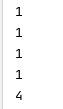# 布局原则

C++的设计者不允许类的大小为0，其原因有很多，比如由它们构成的数组，其大小必然也是0，这会导致指针运算中普遍使用的性质失效。比如，假设类型ZeroSizedT的大小为0，则下面的操作会出现错误：

    ZeroSizedT  z;
auto v =  &z  - &z;  // 计算两个指针或者地址之间的距离


通常而言，上面的差值，一般是用两个地址之间的字节数除以类型大小而得到的，而类型大小为0就不妙了。

虽然不存在0大小的类，但这扇门也没有彻底关死。C++规定，当空类作为基类时，只要不会与同一类型的另一个对象或子对象分配在同一地址，就不需要为其分配任何空间。这个就叫做空基类优化技术。看个例子：

#include <iostream>

class EmptyClass{
};

class EmptyFoo : public EmptyClass{

};
class EmptyThree : public EmptyFoo{

};
int main(){
printf("%lu\n", sizeof(EmptyClass));
printf("%lu\n", sizeof(EmptyFoo));
printf("%lu\n", sizeof(EmptyThree));
}


如果编译器支持空基类优化，上面程序的所有输出结果相同，但是均不为0。也就是说，在类EmptyFoo 中的类 EmptyClass没有分配空间 。 如下图：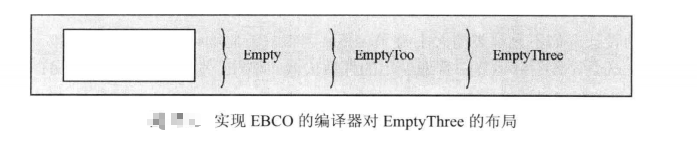如果不支持空基类优化，上面程序的输出结果不同。布局如下图：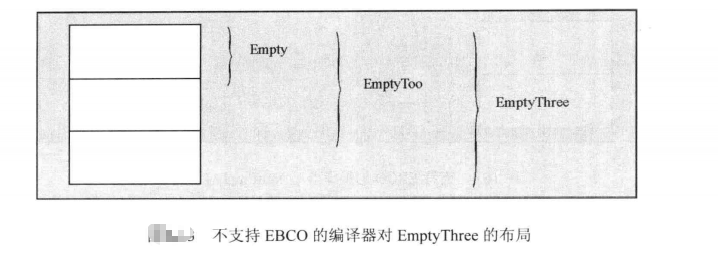再看个例子：

#include <iostream>

class EmptyClass{
};

class EmptyFoo : public EmptyClass{

};
class NoEmpty :public EmptyClass,  public EmptyFoo{

};

int main(){
printf("%lu\n", sizeof(EmptyClass));
printf("%lu\n", sizeof(EmptyFoo));
printf("%lu\n", sizeof(NoEmpty));
}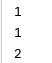NoEmpty 为什么不为空类呢？这是因为NoEmpty 的基类EmptyClass和EmptyFoo 不能分配到同一地址空间，否则EmptyFoo 的基类EmptyClass和NoEmpty 的EmptyClass会撞到同一地址空间上。换句话说，两个相同类型的子对象偏移量相同，这是C++布局规则不允许的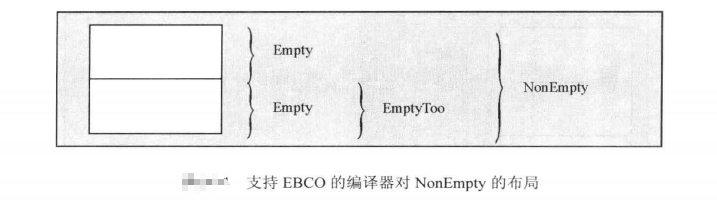对空基类优化进行限制的根据原因在于：我们需要能比较两个指针是否指向同一对象。由于指针几乎总是用地址内部表示，所以我们必须保证两个不同的地址（即两个不同的指针）对应两个不同的对象

# 成员作基类

对于数据成员，则不存在类似空基类优化的技术，否则遇到指向成员的指针时就会出现问题。因此我们可以考虑将成员变量实现为（私有）基类的形式。

在模板中考虑这个问题非常有必要，因为模板参数常常可能是空类；但是对于一般情况，我们并不能依赖这条规则（即模板参数常常可能是基类）；而且如果对某一个模板参数一无所知，也不能很容易就实现空基类优化。看个例子：

template<typename T1, typename T2>
class MyClass{
private:
T1 a;
T2 b;
};


模板参数T1和T2之一或全部，都有可能是空类，那MyClass<T1, T2>就不能得到最优布局，每个这样的实例就可能会浪费一个字的内存。

把模板参数直接作为基类可以解决这个问题：

template<typename T1, typename T2>
class MyClass : private T1, private T2{};


但是，比如当T1和T2是int这样的基本类型时，上面的做法很有问题；另外，当T2和T2类型相同时，也会出问题（这个问题可以用模板特化或者通过添加中间层进行继承的方法[示例：模板的命名参数]解决）；还有一个很大的问题就是增加基类会改变接口；还有一个问题就是继承模板参数甚至能影响到成员函数是否为虚。显然，引入EBCO会引来很多不必要的麻烦

如果一个已知的模板参数的类型必然是类，该模板的另一个成员类型不是空类，那么有一个方法更加可行，大概相反是借助EBCO，把可能为空的类型参数与这个成员”合“起来，比如对于：

template<typename CustomClass>
class Optimizable{
CustomClass info; // 可能为空
void * storage;
};


可以将其改写为：

template<typename CustomClass>
class Optimizable{
private:
BaseMemberPair<CustomClass, void *> info_and_storage;
}


虽然实现可能麻烦了，但是性能可以显著提高
BaseMemberPair 的实现如下：

template<typename Base, typename Member>
class BaseMemberPair : private Base{
private:
Member member;
public:
// 构造函数
BaseMemberPair(Base const & b, Member const & m) : Base(b), member(m) {
}

// 通过first()来访问基类数据
Base const & first() const {
return (Base const  &) *this;
}
Base & first()  {
return (Base   &) *this;
}

// 通过second()来访问基类的成员变量
Member const & second() const {
return this->member;
}
Member & second()  {
return this->member;
}
};


封装在BaseMemberPair中的数据成员(其存储方式在Base为空时可得到优化)，需要通过成员函数first和second访问

展开全文• 我们知道c++中一个空类的大小为1个字节，那么如果一个空类作为基类或者成员对象的时候会怎样呢，是不是还是...然而，基类子对象不受这种制约，而且可以完全从对象布局中被优化掉，若空基类之一亦为首个非静态数据成...

我们知道c++中一个空类的大小为1个字节，那么如果一个空类作为基类或者成员对象的时候会怎样呢，是不是还是一定占用1个字节呢？

c++中为保证同一类型的不同对象地址始终有别，要求任何对象或成员子对象，即使该类型是空的类类型（即没有非静态数据成员的 class 或 struct）也是如此。然而，基类子对象不受这种制约，而且可以完全从对象布局中被优化掉，若空基类之一亦为首个非静态数据成员的类型或其类型的基类，则禁用空基优化，因为要求两个同类型基类子对象在最终派生类型的对象表示中必须拥有不同地址。这种情况的典例是 std::reverse_iterator 的实现（派生自空基类 std::iterator），它持有其底层迭代器（亦派生自 std::iterator）为其首个非静态数据成员。

空基类优化对于标准布局类型 (StandardLayoutType)是被要求的，以维持指向标准布局对象的指针，用 reinterpret_cast 转换后，还指向其首成员，这是标准要求标准布局类型“在同一个类中声明所有非静态数据成员（c++11起 全在派生类或全在某个基类）”，并且“无与首个非静态数据成员同类型的基类”的原因。

#include <cassert>
struct Base {}; // 空类

struct Derived1 : Base {
int i;
};

struct Derived2 : Base {
Base c; // Base，占用 1 字节，后随对 i 的填充
int i;
};

struct Derived3 : Base {
Derived1 c; // 从 Base 派生，占用 sizeof(int) 字节
int i;
};

int main()
{
//TestRank();
// 任何空类类型的对象大小至少为 1
printf("sizeof(Base) = %d \n",sizeof(Base));
// 应用空基优化
printf("sizeof(Derived1) = %d \n",sizeof(Derived1));
// 不应用空基优化
// 基类占用 1 字节，Base 成员占用 1 字节，后随 2 个填充字节以满足 int 的对齐要求
printf("sizeof(Derived2) = %d \n",sizeof(Derived2));
// 不应用空基类优化，
// 基类占用至少 1 字节加填充，以满足首个成员的对齐要求（其对齐要求与 int 相同）
printf("sizeof(Derived3) = %d \n",sizeof(Derived3));
}

运行结果：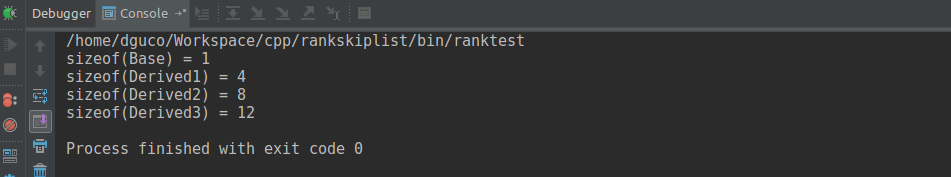我们可以看到除了类Derived1对空基类进行了优化，其他的都没有，证明了上面提到的：若空基类之一亦为首个非静态数据成员的类型或其类型的基类，则禁用空基优化，因为要求两个同类型基类子对象在最终派生类型的对象表示中必须拥有不同地址。

空基类优化常用于具分配器的标准库类（std::vector、std::function、std::shared_ptr 等），使得当分配器无状态时可避免为其分配器成员占用任何额外存储。这是通过将必要的数据成员之一（例如 vector 的 begin、end 或 capacity 指针）与分配器一起，在 boost::compressed_pair 的某种等价物中存储而实现的。

#include <iostream>

struct Empty {}; // 空类

struct X {
int i;
Empty e;
};

struct Y {
int i;
};

struct Z {
char c;
};

struct W {
char c;
};

int main()
{
// 任何空类类型对象的大小至少为 1
static_assert(sizeof(Empty) >= 1);

// 至少需要多一个字节以给 e 唯一地址
static_assert(sizeof(X) >= sizeof(int) + 1);

// 优化掉空成员
std::cout << "sizeof(Y) == sizeof(int) is " << std::boolalpha
<< (sizeof(Y) == sizeof(int)) << '\n';

// e1 与 e2 不能共享同一地址，因为它们拥有相同类型，尽管它们标记有 [[no_unique_address]]。
// 然而，其中一者可以与 c 共享地址。
static_assert(sizeof(Z) >= 2);

// e1 与 e2 不能拥有同一地址，但它们之一能与 c 共享，而另一者与 c 共享
std::cout << "sizeof(W) == 2 is " << (sizeof(W) == 2) << '\n';
}

输出（注意 需支持c++20的编译器才会得到正确的输出，这里我也是截的网上的结果）：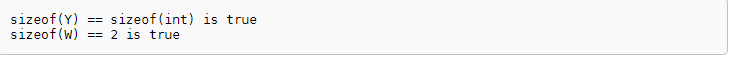展开全文• 0.写作目的 好记性不如烂笔头。 总结： 在VS2012中(win32) ...在派生类中，如果只存在继承一个空基类，而没有在派生类中定义该空基类或者其他空基类的对象作为成员数据，则虽然sizeof(空基类) ...

# 0.写作目的

好记性不如烂笔头。

总结：

在VS2012中(win32)

1) 在多继承中，只存在一个“空类”的继承，这里的空类只是不包括non-static成员变量，空类中可以包括enums，typedef，static，non-virtual函数。

在派生类中，如果只存在继承一个空基类，而没有在派生类中定义该空基类或者其他空基类的对象作为成员数据，则虽然sizeof(空基类) = 1，但是由于空基类优化EBO，因此在计算派生类的sizeof时，不会计算继承的空基类的大小。

在派生类中，只继承一个空基类，但是在派生类中定义了该空基类或者其他空基类的对象作为成员数据，如果只定义一个空基类对象，则占用一个字节，但是由于EBO，在计算派生类的大小时，采样对其的方式，即32位对齐，则派生类的大小会多出3个字节。如果定义多个空基类数据成员，则4个空基类成员构成一个32位，对于剩下的空基类向上取整，凑出一个32位。且继承基类的地址，按照继承声明的顺序（如下面的代码中，地址在b2, b1的地址之后）。

2) 在多继承中，存在多个空基类的继承时，只有最后一个空基类的继承不分配空间，其余继承的空基类均分配一个字节。对于在派生类中定义的空基类的对象，每个对象分配一个字节。然后进行EBO，不足4个字节的向上取整。

在Ubuntu16 g++5.4.0中

无论多继承中，存在多少个空基类的继承，空基类的继承均不分配空间，而且空间类的地址与派生类的对象首地址相同，这与VS2012(win32)中不同。然后对派生类中的空基类对象，每个空基类对象分配一个字节，然后进行EBO，不足4个字节的向上取整。

（注意： 多继承中，先对继承的对象进行构造，然后对派生类中基类的对象进行构造，最后执行派生类的构造函数体中的代码。便于理解下面构造函数的结果。）

# 1. 多继承中，存在一个空基类，派生类的大小计算

#include<iostream>

using namespace std;

class B1
{
protected:
int b1;
public:
B1(int i)
{ b1 = i;
cout<<"this = "<<this<<" Constructing B1"
<<" &this->b1="<<&b1<<" this->b1="<<b1<<endl;}
~B1(){
cout<<"this = "<<this<<" Deconstructor B1"<<endl;
}
};
class B2
{
protected:
int b2;
public:
B2(int j)
{b2 = j;
cout<<"this = "<<this<<" Constructing B2"
<<" &this->b2="<<&b2<<" this->b2="<<b2<<endl;}
~B2(){
cout<<"this = "<<this<<" Deconstructor B2"<<endl;
}
};
class B3
{
//int b3;
public:
B3()
{ //b3 = 0;
cout<<"this = "<<this<<" Constructing B3"<<" this="<<this
<<endl;}
~B3(){
cout<<"this = "<<this<<" Deconstructor B3"<<endl;
}
};

class B4{
public:
B4(){
cout<<"this = "<<this<<" Constructing B4"<<" this="<<this
<<endl;
}
~B4(){
cout<<"this = "<<this<<" Deconstructor B4"<<endl;
}
};
class D : public B2,public B1,public B3
{
public:
/*C(int a, int b, int c, int d)
:B1(a), memB2(d), memB1(c),    B2(b) {
cout<<"this = "<<this << " Constructor C"<<endl;
}*/
D(int a, int b, int c, int d)
:B1(a),    B2(b) {
cout<<"this = "<<this << " Constructor D"<<endl;
}
~D(){
cout<<"this = "<<this<<" DeConstructor D"<<endl;
}
void print(){
cout<<"***************************************"<<endl;
cout<<"\tD: this="<<this<<endl;
cout<<"\tD: &this->b1" <<&b1<<" this->b1="<<b1<<endl;
cout<<"\tD: &this->b2" <<&b2<<" this->b2="<<b2<<endl;
//cout<<"\tC: &this->memB1=" <<&memB1<<endl;
//cout<<"\tC: &this->memB2=" <<&memB2<<endl;
cout<<"\tD: &this->memB3="<<&memB3<<endl;
cout<<"\tD: &this->memB3_2="<<&memB3_2<<endl;
cout<<"\tD: &this->memB4_1="<<&memB4_1<<endl;
cout<<"\tD: &this->memB4_2="<<&memB4_2<<endl;
cout<<"\tD: &this->memB3_5="<<&memB3_5<<endl;
cout<<"\tD: &this->dd="<<&dd<<endl;
cout<<"\tD: sizeof(D)="<<sizeof(*this)<<endl;
//cout<<"\tC: sizeof(B1)="<<sizeof(memB1)<<endl;
//cout<<"\tC: sizeof(B2)="<<sizeof(memB2)<<endl;
cout<<"\tD: sizeof(B3)="<<sizeof(memB3)<<endl;
}
private:
//B1 memB1;
//B2 memB2;
B3 memB3;
B3 memB3_2;
B4 memB4_1;
B4 memB4_2;
B3 memB3_5;
int dd;
};

int main()
{

{
D d(5, 6, 7, 8);
d.print();
cout<<"sizeof(d)="<<sizeof(d)<<endl;
}
system("pause");
return 0;
}



VS2012(win32)结果：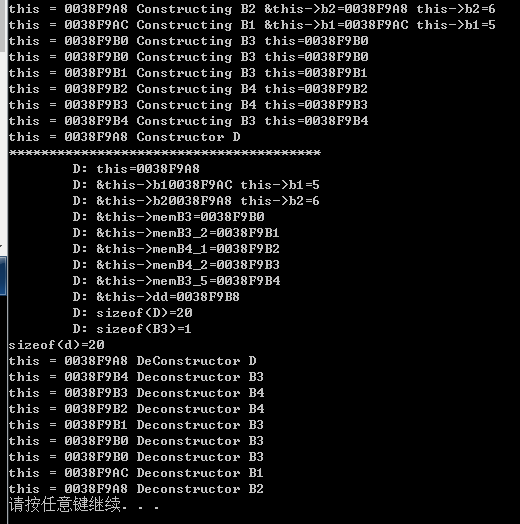由上图得到，派生类D的对象d的内存分配情况：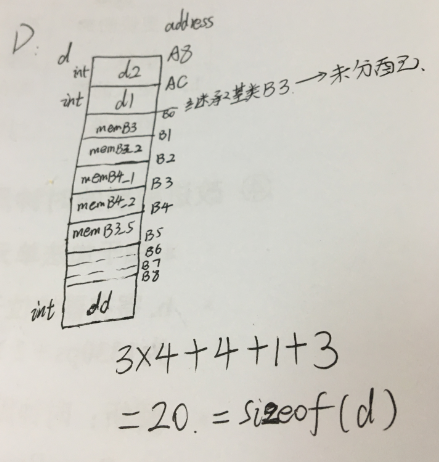在Ubuntu16 g++ 5.4.0中，继承的空基类的地址与派生类对象的首地址相同。

# 2. 多继承中，存在两个空基类，派生类的大小计算

#include<iostream>

using namespace std;

class B1
{
protected:
int b1;
public:
B1(int i)
{ b1 = i;
cout<<"this = "<<this<<" Constructing B1"
<<" &this->b1="<<&b1<<" this->b1="<<b1<<endl;}
~B1(){
cout<<"this = "<<this<<" Deconstructor B1"<<endl;
}
};
class B2
{
protected:
int b2;
public:
B2(int j)
{b2 = j;
cout<<"this = "<<this<<" Constructing B2"
<<" &this->b2="<<&b2<<" this->b2="<<b2<<endl;}
~B2(){
cout<<"this = "<<this<<" Deconstructor B2"<<endl;
}
};
class B3
{
//int b3;
public:
B3()
{ //b3 = 0;
cout<<"this = "<<this<<" Constructing B3"<<" this="<<this
<<endl;}
~B3(){
cout<<"this = "<<this<<" Deconstructor B3"<<endl;
}
};

class B4{
public:
B4(){
cout<<"this = "<<this<<" Constructing B4"<<" this="<<this
<<endl;
}
~B4(){
cout<<"this = "<<this<<" Deconstructor B4"<<endl;
}
};

class C : public B2,public B1,public B3, public B4
{
public:
/*C(int a, int b, int c, int d)
:B1(a), memB2(d), memB1(c),    B2(b) {
cout<<"this = "<<this << " Constructor C"<<endl;
}*/
C(int a, int b, int c, int d)
:B1(a),    B2(b) {
cout<<"this = "<<this << " Constructor C"<<endl;
}
~C(){
cout<<"this = "<<this<<" DeConstructor C"<<endl;
}
void print(){
cout<<"***************************************"<<endl;
cout<<"\tC: this="<<this<<endl;
cout<<"\tC: &this->b1" <<&b1<<" this->b1="<<b1<<endl;
cout<<"\tC: &this->b2" <<&b2<<" this->b2="<<b2<<endl;
//cout<<"\tC: &this->memB1=" <<&memB1<<endl;
//cout<<"\tC: &this->memB2=" <<&memB2<<endl;
cout<<"\tC: &this->memB3="<<&memB3<<endl;
cout<<"\tC:: &this->memB3_2="<<&memB3_2<<endl;
cout<<"\tC: &this->memB4="<<&memB4<<endl;
cout<<"\tC: &this->memB4_2="<<&memB4_2<<endl;
cout<<"\tC: &this->cc="<<&cc<<endl;
cout<<"\tC: sizeof(C)="<<sizeof(*this)<<endl;
//cout<<"\tC: sizeof(B1)="<<sizeof(memB1)<<endl;
//cout<<"\tC: sizeof(B2)="<<sizeof(memB2)<<endl;
cout<<"\tC: sizeof(B3)="<<sizeof(memB3)<<endl;
cout<<"\tC: sizeof(B3)="<<sizeof(B3)<<endl;
cout<<"\tC: sizeof(B4)="<<sizeof(B4)<<endl;
}
private:
//B1 memB1;
//B2 memB2;
B3 memB3;
B3 memB3_2;
B4 memB4;
B4 memB4_2;
int cc;
};
int main()
{
{
C c(1, 2, 3, 4);
c.print();
cout<<"sizoef(c)="<<sizeof(c)<<endl;
}
system("pause");
return 0;
}


VS2012(win32)运行结果（ubuntu g++ 5.4.0的运行结果不同）：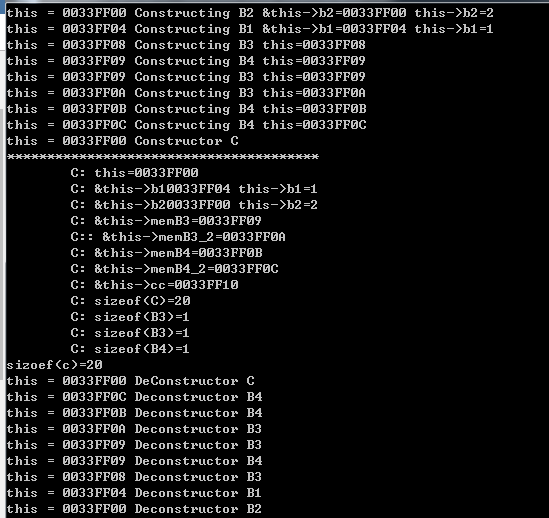VS2012(win32)和ubuntu g++ 5.4.0派生类C的对象c的内存分布：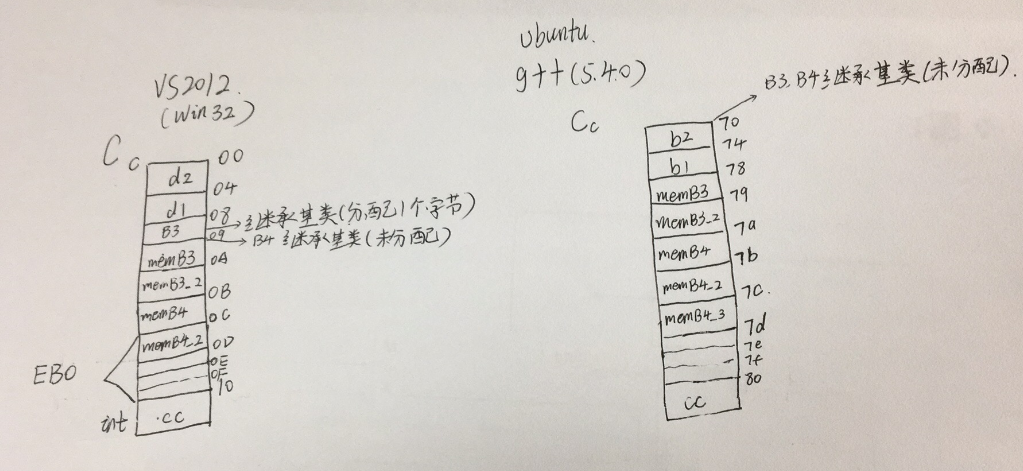# 3. 多继承中，存在三个空基类，派生类的大小计算

直接给出结果(VS2012(win32))：(前两个继承的空基类各分配了一个字节，第3个继承空基类没有分配空间，派生类中定义的空基类的对象，均分配了一个字节，然后在计算派生类大小时，不足4个字节的进行上取整补全。)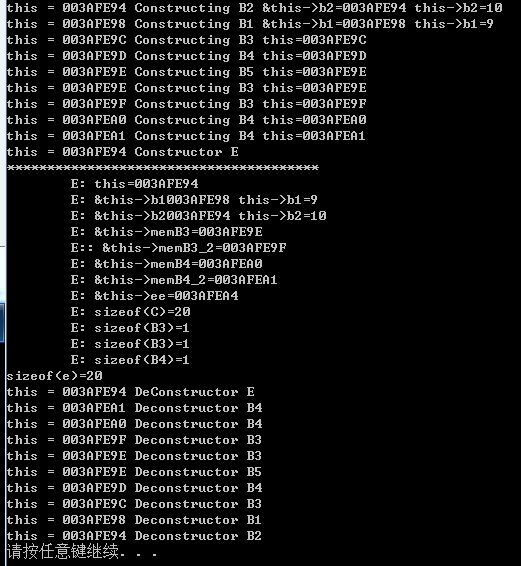ubuntu16 g++5.4.0中，派生类中的空基类均不分配空间，且继承的空基类的地址与派生类对象的首地址相同。

There may be some mistakes in this blog. So, any suggestions and comments are welcome!

# [Reference]

展开全文C++
•c++ 开发语言
• 允许基类子对象大小为零。 解释 为保证同一类型的不同对象地址始终有别，要求任何对象或成员子对象（除非为[[no_...然而，基类子对象不受这种制约，而且可以完全从对象布局中被优化掉： #include <cas...
• STL设计之EBO空基类优化导语空类空基类优化STL中的EBO世界利用EBO,手动实现一个简单的内存分配与释放 导语 EBO简称Empty Base Optimization。 本节从空类开始，到STL内部，到测试，再到我们自己实现一个EBO，对比...
• 1、C语言中的结构体 曾对C语言中的结构体进行了简单分析（直达）   2、类对象的大小 下面输出什么？ #include &lt;iostream&gt; using namespace std; class Base { }; int main() { ...
• 空基类：类----在运行期内部表示不耗费内存； 即类只包含 非虚成员函数 静态数据成员 （非静态数据，虚函数，虚基类运行期...就不需为其分配任何空间=====空基类优化 注：BOOST中的compressed_pair使用此原理。
• 空基类优化EBO之深度探索 继承情景  我们知道一个空的类，也就是其内部没有非静态数据成员，没有虚指针（包括指向虚函数表和虚基类子对象的指针），它的大小通常为1，当然在某些对齐要求严格系统上可能是另一个数...C++
• 结构选择，将类结构的可变部分放入公共基类基类目标并使用一个元程序在它们之间选择。 #include <type_traits> using namespace std; template<typename F, bool F_empty, typename G, bool G_...c++ 开发语言
• 参考文章 虚继承与空基类优化 。 class TEST_X {}; // 1 class TEST_Y : public virtual TEST_X { // 12 virtual void fun() {}; // 虚表4 //指虚基类的表指针4 char c; }; // 字节对齐 4 // 空基类...c++ 开发语言 后端
• 对于c++中的一个类  class X { };  事实上并不是的，sizeof(X)并不等于0， 一般的结果是1。每个X的对象都有一个隐晦的1 bytes，是被编译器安插进去的一个char，这样可以使得这个class的两个objects在...c++ 编译器 sizeof
• STL 设计之 EBO(空基类优化)0.导语EBO 简称 Empty Base Optimization。本节从空类开始，到 STL 内部，到测试，再到我们自己实现一个 ...
• 1、概念 C++中有“空”类的概念，这就意味着在运行期间其内部不好任何内存。 只包含类型的成员函数、...2、空基类优化如下: #include<iostream> using namespace std; class Empty{ typedef int Int; ......